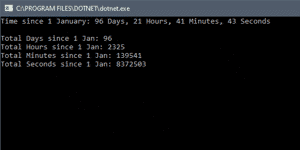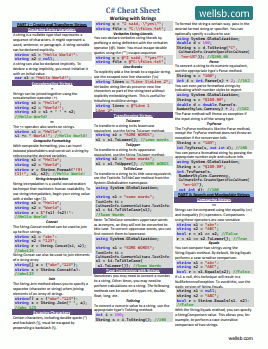# C# DateTime Subtract

## COUNT DAYS BETWEEN TWO DATES USING DATETIME.SUBTRACT

by

Suppose you want to get the number of days between two dates, or the number of seconds between two times. In C#, you can use the `DateTime.Subtract` method to compute the difference between dates and times.

## DateTime.Subtract Method

The `DateTime.Subtract` method will determine the duration between two dates or times. More specifically, it will return a `TimeSpan` object which represents the difference between two `DateTime` objects.

The syntax for using `DateTime.Subtract` follows:

``````TimeSpan timeDifference = recentDate.Subtract(oldDate);
``````

In this case, the date represented by `oldDate` will be subtracted from `recentDate` and the difference will be stored in the `timeDifference` variable.

## The TimeSpan Object

A `TimeSpan` object represents an interval between two dates and stores the difference in Days, Hours, Minutes, Seconds, and Milliseconds. Let us consider an example where we are interested in determining the amount of time that has passed between New Years Day and the current instance of time.

``````DateTime currentDateTime = DateTime.Now;
DateTime newYear = new DateTime(DateTime.Now.Year, 1, 1);

TimeSpan sinceNewYear = currentDateTime.Subtract(newYear);

Console.WriteLine(\$"Time since 1 January: {sinceNewYear.Days} Days, " +
\$"{sinceNewYear.Hours} Hours, {sinceNewYear.Minutes} Minutes, " +
\$"{sinceNewYear.Seconds} Seconds");
``````

On Line 1, I have declared a new DateTime object and initialized it with the current date and time using `DateTime.Now`.

On Line 2, I created a new DateTime object and assigned it the value of January 1 of the current year. This constructor type takes integer values representing the year, month, and day and saves it as a new DateTime object. For example, `DateTime newYear = new DateTime(2019, 1, 1);` would initialize a DateTime instance representing January 1, 2019.

On Line 4, I have created a TimeSpan object that represents the time difference between `currentDateTime` and `newYear`. This is functionally equivalent to the following:

``````TimeSpan diff = currentDateTime - newYear;
``````

## TimeSpan Properties

The `TimeSpan` object contains other properties which give the interval as different time units. The TotalDays, TotalHours, TotalMinutes, TotalSeconds, and TotalMilliseconds properties represent the span of time, in each respective unit, as a decimal number.

``````DateTime currentDateTime = DateTime.Now;
DateTime newYear = new DateTime(DateTime.Now.Year, 1, 1);

TimeSpan sinceNewYear = currentDateTime.Subtract(newYear);

Console.WriteLine(\$"Time since 1 January: {sinceNewYear.Days} Days, " +
\$"{sinceNewYear.Hours} Hours, {sinceNewYear.Minutes} Minutes, " +
\$"{sinceNewYear.Seconds} Seconds");

Console.WriteLine();
Console.WriteLine(\$"Total Days since 1 Jan: {sinceNewYear.TotalDays}");
Console.WriteLine(\$"Total Hours since 1 Jan: {sinceNewYear.TotalHours}");
Console.WriteLine(\$"Total Minutes since 1 Jan: {sinceNewYear.TotalMinutes}");
Console.WriteLine(\$"Total Seconds since 1 Jan: {sinceNewYear.TotalSeconds}");
``````

On Line 11-14, I accessed various properties of the sinceNewYear TimeSpan object to represent the same time interval in days, hours, minutes, and seconds.

If you are only interested in whole number values, you can use `Math.Truncate()` to remove the fractional elements.## The Bottom Line

In this tutorial, you learned how to subtract two dates in C#. This technique can be useful for triggering an action after a designated number of days has elapsed. It can also be used to break out of a loop after three seconds. Still have questions about this technique? Let me know in the comments.

#### Don't stop learning!

There is so much to discover about C#. That's why I am making my favorite tips and tricks available for free. Enter your email address below to become a better .NET developer.

#### Did you know?Our beautiful, multi-column C# reference guides contain more than 150 tips and examples to make it even easier to write better code.